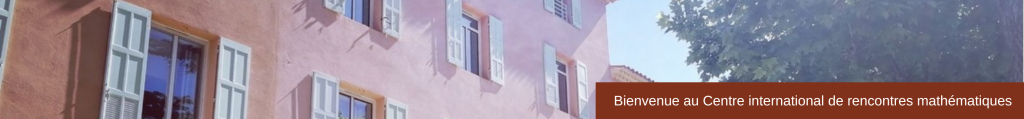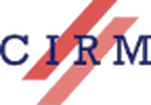THEMATIC MONTH 2016
Week 2: Mathematical Statistics and Inverse Problems
February 8 – 12, 2016
 The main goal in this week is to bring together leading researchers in the area of mathematical statistics and inverse problems in order to exchange the ideas and initialize new researches.The workshop will be focusing on the following topics: regularization of ill-posed inverse problems, density deconvolution, quantum statistics, multivariate structural functional estimation and detection} along with applications related to econometrics, tomography and astrophysics. During the workshop three  mini-courses will be given: Cristina Butucea (Université Paris Est-Marne la Vallée Quantum statistical models and inference. Jean-Pierre Florens (Université Toulouse1)  Inverse problems in econometrics: examples and specific theoretical problems. Gerard Kerkyacharian (Université Pierre et Marie Curie) Geometry and inverse problems. Example tomography and astrophysics. Scientific Committee Oleg Lepski (Aix-Marseille Université)Dominique Picard (Université Paris Diderot) Organizing Committee Florent Autin (Aix-Marseille Université)Yuri Golubev (Aix-Marseille Université)Christophe Pouet (Ecole Centrale Marseille) Speakers Butucea Cristina (Université Paris Est-Marne la Vallée) Quantum statistical models and inference Buzun Nazar  (WIAS Berlin) Multiplier bootstrap for change point detection Dalalyan Arnak (ENSAE Paristech) Convex programming approach to robust estimation of a multivariateGaussian model Enikeeva Farida (Université de Poitiers) Bump detection in a heterogeneous Gaussian regression Ermakov Mikhail (IPME RASc St.Petersburg) On consistent hypothesis testing Florens Jean-Pierre (Université Toulouse 1) Inverse problems in econometrics: examples and specific theoretical prob-lems Jean-Marc Freymuth (University of Cambridge) Minimax optimal detection of structure for multivariate data Goldenshluger Alexandre (Tel Aviv University) The M/G/infinit estimation problem revisited Hengartner Nicolas (Los Alamos National Laboratory) Denoising nonlinear dynamical systems Hohage Thorsten (Göttingen University) Variational Regularization of Nonlinear Statistical Inverse Problems Huckemann Stephan (Göttingen University) Drift estimation in sparse sequential dynamic imaging Ibragimov Ildar (Steklov Mathematical Institute) Estimation of in nite-dimensional parameter in lp spaces Jan Johannes (CREST-Ensai and Université catholique de Louvain) Adaptive Bayesian estimation in indirect Gaussian sequence space models Kerkyacharian Gérard (Université Paris 10) Geometry and inverse problems. Example tomography and astrophysics Lepski Oleg (Aix-Marseille Université) Adaptive Estimation in the Convolution Structure Density Model Marteau Clément (INSA Toulouse, France) Minimax goodness-of- t testing in ill-posed inverse problems with partially unknown operators Mathé Peter (Weierstrass Institute, Germany)   Discrepancy based model selection in statistical inverse problems Matzner-Loeber Eric (Université Rennes 2) Nonparametric admissible estimator Munk Axel (Göttingen University) Statistical Blind Source Separation Pensky Marianna (University of Central Florida) Laplace deconvolution and its application to the analysis of dynamiccontrast enhanced imaging data Pereversev Sergei (Johann Radon Institute, Austria)) Aggregation of regularized rankers by means of a linear functional strategy Reiss Markus (Humboldt University Berlin) From prediction error to estimation error bounds ﻿Tsybakov Alexandre﻿ (CREST-ENSAE,  Ecole polytechnique) Sharp minimax and adaptive variable selection